## Symbols & Notations TYPE-I (ii) Questions based on figures & mathematical operations

• Post author:
• Post category:Blog

Symbols & Notations

TYPE-I (ii) Questions based on figures & mathematical operations

1. (SSC CPO Sub-Inspector Exam. 03.09.2006) (SSC Combined Matric Level (PRE) Exam. 21.05.2000 (Ist Sitting (East Zone) (SSC CGL Tier-I (CBE) Exam.11.09.2016) (Ist Sitting)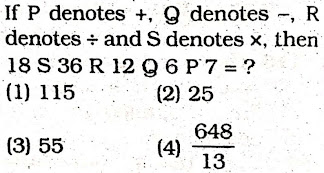2. (SSC CISF Constable (GD) Exam. 05.06.2011)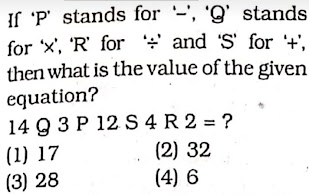3. If A stands for ‘addition’, M for ‘multiplication’, D for ‘division’, G for ‘greater than’ and L for “Lesser than’ then which of the following will be logically correct?

(1) 20A 4D 4L 4A 6D2

(2) 20 D 5G,8D 4A 6M3

(3) 20D 4A 4L 4A 2M3

(4) 20A 2G 10M 3A 12D 2

FCI Assistant Grade-III Exam. 05.02.2012 (Paper-I) East Zone (IInd Sitting)

4. FCI Assistant Grade-III Exam. 25.02.2012 (Paper-I) North Zone (Ist

Sitting)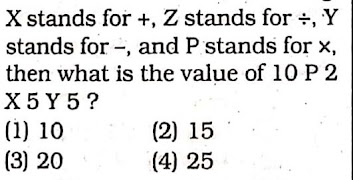5. (SSC Graduate Level Tier-I Exam. 21.04.2013, IInd Sitting)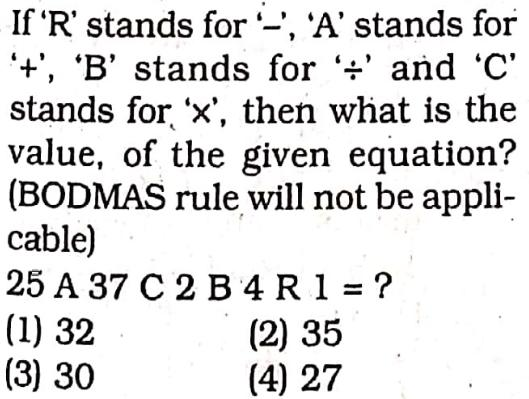6.(SSC Graduate Level Tier-I Exam. 19.05.2013, Ist Sitting)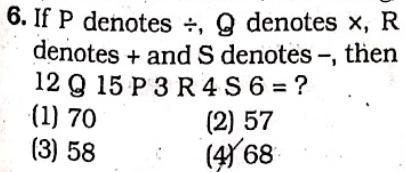7. If ‘P’ denotes ‘multiplied by’, T denotes ‘subtracted from’, ‘M’ denotes ‘added to’ and ‘B’ denotes ‘divided by then what should be the correct response of: 12 P 6 M 15 T 16 B 4?

(1) 70

(2) 75

(3) 83

(4) 110

(SSC Graduate Level Tier-I Exam. 19.05.2013, IInd Sitting)